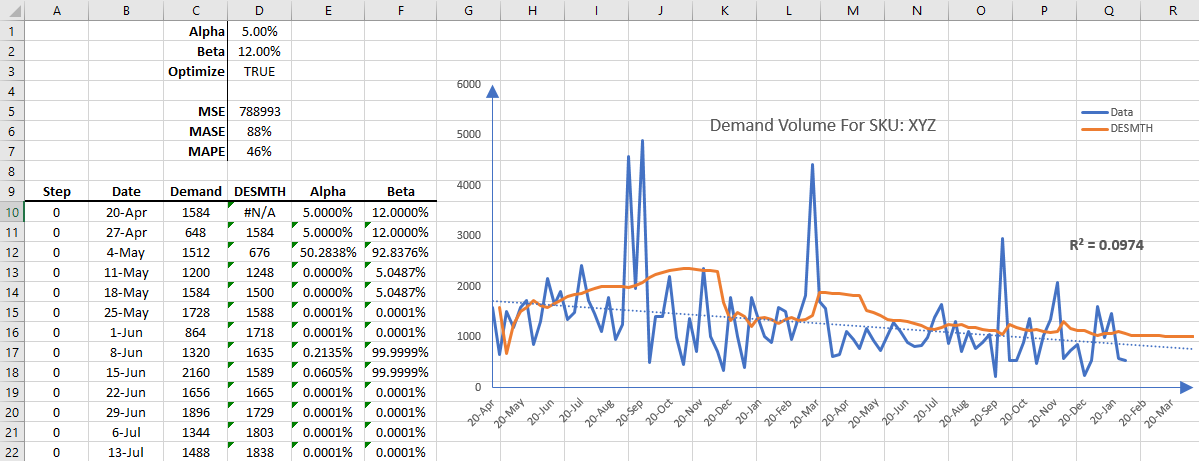# Suavización Exponencial Doble de Holt en Excel (DESMTH)

Échenle un vistazo a nuestro tutorial de Suavizado Exponencial Doble de Holt. La lista de reproducción contiene tutoriales que les enseñarán cómo utilizar el botón de optimización y calcular con decomposición estacional.

Optimization On Optimization Off Components

## Optimization On

Este video les mostramos cómo usar la Función de suavizado exponencial doble de Holt en NumXL con un botón de optimización para factores de suavizado.

Guion de Video

Scene 1:

Hello and welcome to the exponential smoothing tutorial series. In this tutorial we'll resume the demonstration of the double exponential smoothing function and use the built-in optimizer in NumXL.

For the sample data we are using the same weekly demand volume data of a hypothetical product. Let's begin by selecting cell D10.

Scene 2:

Examine the self formula in the formula toolbar. Notice we already have a call for the double exponential smoothing function so press F2 to edit.

Click on the FX button located on the left side of the equation toolbar this will invoke the function arguments dialog box for the double exponential smoothing function.

Scene 3:

Notice that the cell D3 is used for the optimize argument so we don't need to change the formula, just the value in D3 to turn on the optimizer. Now click cancel to return to your worksheet.

Scene 4:

Select cell D3, then type in true or 1 then hit enter when done.

Scene 5:

Since we have the automatic calculation on, all the values of the smooth time series are recalculated using optimal smoothing factors known as alpha and beta. Notice the favorable changes in mean squared error, mean absolute scaled error and symmetric mean absolute percentage error.

The calibrated double exponential smoothing has a 22% lower a mean absolute error than that of a naive reference model. So what about alpha value in D1 and beta value in D2? When the optimizer flag is turned on the function uses a value of alpha as a starting value for the optimizer.

Scene 5:

The double exponential smoothing function calculates the optimal values for beta and alpha using the available information or data. The available data increases the time so the function calculates a new value for each step. Let's examine the values of those parameters, so select the cell E10. Start typing the double exponential smoothing function, =DESMTH(. When the function is found click on the FX button found on the left side of the equation toolbar.

Scene 6:

This will invoke the function arguments dialog box for the double exponential smoothing function.

Scene 7:

Select the input cell range, this is the same cell range we used earlier for the forecast in column D and then lock the cell reference by pressing F4.

Scene 8:

Enter a value of true or one in the order field. For the initial value of alpha let's use the value in D1 and unlock the cell by pressing F4.

Scene 9:

For the initial beta value let's use the value in D2 and lock it by pressing F4. For the optimize switch, let's use the value in cell D3 and then lock the cell reference by pressing F4. Set the forecast time to zero, or set it to the value in cell A10 and then lock the cell for column movement.

For return type, type in one, for returning the value of the level smoothing parameter alpha and then click OK.

Scene 10:

The built-in optimizer requires a few non-missing observations to run or it will return the same starting value alpha, as it is the case here.

Scene 11:

Let's repeat the same procedure for beta. Select the cell in F10 and start typing =DESMTH(, and when a function is found, click on the FX button found on the left side of the equation toolbar.

Scene 12:

Use the same input we used earlier for all arguments except for return type. For return type, type in two for returning the value of the transmitting parameter beta and then click OK.

Scene 13:

Similar to alpha, the built-in optimizer requires a few non-missing observations to run or it will return the same starting value as is the case here. Select E10 and F10 cell range. So copy the formula to the cells below it.

Scene 14:

The values of the smoothing parameters at each period is calculated, so let's plot the values of the level smoothing parameter versus time.

The graph for alpha shows some volatility of the computed alpha value throughout the sample in the first year. In the second year the value of the smoothing factor is relatively stable.

So what's going on? The optimal user finds optimal values by minimizing the SSE, in our case the SSE function seems to have multiple minima and their values are comparably close. Earlier in the sample data the first minima seemed to have a lower value and as we have more observations, the second minima have a lower value, so it was picked up by the optimizer.

So which alpha do we use? Well we prefer a stable alpha over time so we could designate a part of the sample as a training set and use the rest to assess the forecasting power.

Scene 15:

Let's plot the value of the transmitting factor versus time. The graph for alpha shows some volatility of the computed beta value throughout the sample in the first year. In the second year the value of the smoothing factor is relatively stable and equal to zero.

In a follow-up tutorial we'll decompose the input data into two components, level and trend, and query the double exponential function for their values.Scene 16:

That's all for now, thank you for watching!

### Comentarios

El artículo está cerrado para comentarios.

¿Fue útil este artículo?
Usuarios a los que les pareció útil: 0 de 1